#### 期刊菜单

A Novel Dynamic Analysis Method of Shale Gas Reservoir
DOI: 10.12677/APF.2021.111002, PDF, HTML, XML, 下载: 194  浏览: 564  国家科技经费支持

Abstract: In order to realize commercial development, multi-stage fracturing and horizontal well technologies are often used in tight gas reservoir. At present, there are mainly two methods for tight gas reser-voir performance analysis: analytical method and numerical simulation method. The analytical method is fast, but it is only suitable for homogeneous reservoir. The numerical simulation method can accurately calculate complex geometry wells (such as fractured horizontal wells) in heteroge-neous reservoir, but it is time-consuming and laborious. In order to improve the accuracy and effi-ciency of dynamic analysis of tight gas reservoir, a semi analytical method for dynamic analysis of multiple fractured horizontal wells in tight gas reservoir, namely fast marching method (FMM) is proposed. Firstly, considering the high compressibility of gas, the diffusion equation of tight gas is established. Then, fast marching method is proposed to solve the propagation time of pressure wave, and combined with the geometric approximation method, the relationship between the re-lease volume and time is quantified. Then, the commercial numerical simulation software CMG is used to verify the accuracy and reliability of the new method. The results show that the dynamic analysis method based on fast marching method is more accurate than the conventional dynamic analysis method, and the calculation speed is increased by more than 10% compared with the commercial software. Finally, the working process of fast marching method to carry out the dynamic analysis of fractured horizontal wells in tight gas reservoirs is described. It has good application value for the dynamic analysis of tight gas reservoirs with simple structural conditions.

1. 引言

2. 数学模型

2.1. 扩散方程的建立

$\nabla \cdot \left[\frac{k\left(\stackrel{\to }{x}\right)}{\mu \left(p\right)Z\left(p\right)}p\nabla p\right]=\varphi \frac{\partial }{\partial t}\left[\frac{p}{Z\left(p\right)}\right]$ (1)

${C}_{\text{t}}\left(p\right)=\frac{1}{\rho }\frac{\text{d}\rho }{\text{d}p}=\frac{Z\left(p\right)}{p}\frac{\text{d}}{\text{d}p}\left[\frac{p}{Z\left(p\right)}\right]$ (2)

$\frac{\text{d}}{\text{d}}\left[\frac{p}{Z\left(p\right)}\right]=\frac{{C}_{\text{t}}\left(p\right)p}{Z\left(p\right)}\frac{\text{d}p}{d}$ (3)

$m\left(p\right)={\left(\frac{{\mu }_{\text{g}}Z}{p}\right)}_{i}\underset{0}{\overset{p}{\int }}\frac{p\text{d}p}{{\mu }_{\text{g}}Z}$ (4)

${t}_{\text{p}}={\left({\mu }_{\text{g}}{c}_{\text{t}}\right)}_{i}\underset{0}{\overset{t}{\int }}\frac{\text{d}t}{{\mu }_{\text{g}}{C}_{\text{t}}}$ (5)

$\nabla \cdot \left[k\left(\stackrel{\to }{x}\right)\cdot \nabla m\left(\stackrel{\to }{x},{t}_{\text{p}}\right)\right]=\varphi \cdot {\left[\mu \left(p\right){C}_{\text{t}}\left(p\right)\right]}_{i}\frac{\partial m\left(\stackrel{\to }{x},{t}_{\text{p}}\right)}{\partial {t}_{\text{p}}}$ (6)

$\varphi \cdot {\left[\mu \left(p\right){C}_{\text{t}}\left(p\right)\right]}_{i}\cdot \left(-i\omega \right)\stackrel{¯}{m}\left(\stackrel{\to }{x},\omega \right)=k\left(\stackrel{\to }{x}\right){\nabla }^{2}\stackrel{¯}{m}\left(\stackrel{\to }{x},\omega \right)+\nabla k\left(\stackrel{\to }{x}\right)\cdot \nabla \stackrel{¯}{m}\left(\stackrel{\to }{x},\omega \right)$ (7)

$\stackrel{¯}{m}\left(\stackrel{\to }{x},\omega \right)={\text{e}}^{-\sqrt{-i\omega }\cdot \tau \left(\stackrel{\to }{x}\right)}\underset{k=0}{\overset{\infty }{\sum }}\frac{{C}_{k}\left(\stackrel{\to }{x}\right)}{{\left(\sqrt{-i\omega }\right)}^{k}}$ (8)

$\sqrt{\alpha \left(\stackrel{\to }{x}\right)}\cdot |\nabla \tau \left(\stackrel{\to }{x}\right)|=1$ (9)

$\alpha \left(\stackrel{\to }{x}\right)=\frac{k\left(\stackrel{\to }{x}\right)}{\varphi \cdot {\left[\mu \left(p\right){C}_{\text{t}}\left(p\right)\right]}_{i}}$ (10)

2.2. 压力波传播时间的求解

$\mathrm{max}{\left({D}_{ij}^{x}\tau ,{D}_{ij}^{+x}\tau ,0\right)}^{2}+\mathrm{max}{\left({D}_{ij}^{y}\tau ,{D}_{ij}^{+y}\tau ,0\right)}^{2}=\frac{1}{\alpha }$ (11)(a)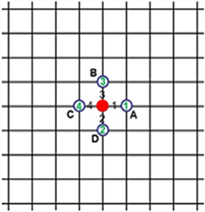(b)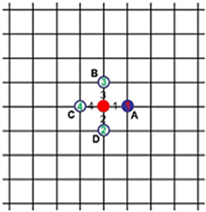(c)(d)(e)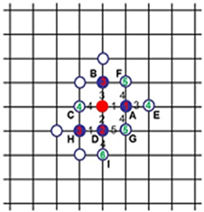(f)

Figure 1. Schematic diagram of FMM calculation in Cartesian coordinate grid. (a) Marking the starting point of pressure wave propagation; (b) Marking the adjacent unknown node; (c) Marking the second step pressure wave propagation of point A; (d) Marking a point adjacent to the unknown node; (e) Marking the third step pressure wave propagation point D; (f) Marking D and H points adjacent to the unknown node

$t=\frac{{\tau }^{2}}{2}\frac{\text{d}\mathrm{ln}\tau }{\text{d}\mathrm{ln}{V}_{\text{p}}\left(\tau \right)}$ (12)

2.3. 致密气井生产动态分析

$\delta p\cong \delta \stackrel{¯}{p}=-\frac{1}{{C}_{\text{t}}}\frac{{q}_{\text{well}}\delta t}{{V}_{\text{p}}\left(t\right)}$ (13)

${q}_{\text{g}}\left({t}_{\text{p}}\right)=\frac{{T}_{\text{sc}}}{T{p}_{\text{sc}}}\cdot {\left(\frac{p}{{\mu }_{\text{g}}Z}\right)}_{i}\cdot \frac{m\left({p}_{i}\right)-m\left(p\right)}{\underset{0}{\overset{{V}_{\text{p}}\left(t\right)}{\int }}\frac{1}{k\cdot \varphi \cdot {A}^{2}}\left(1-\frac{{V}_{\text{p}}}{{V}_{\text{p}}\left({t}_{\text{p}}\right)}\right)\cdot \text{d}{V}_{\text{p}}}$ (14)

3. 模型验证

3.1. 物理模型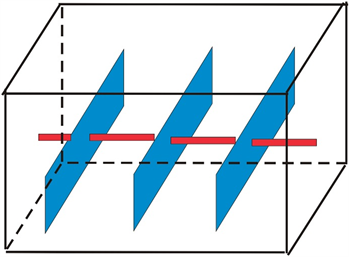Figure 2. Schematic diagram of tight gas multistage fracturing horizontal well modelTable 1. Basic reservoir parameters of fractured horizontal wells in tight gas reservoirs

3.2. 模型验证

4. 生产动态分析工作流程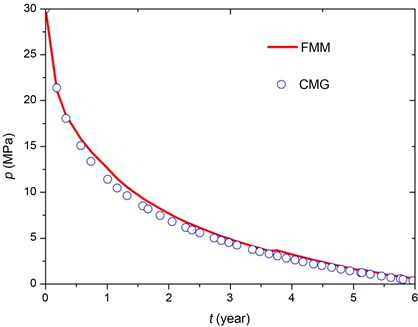Figure 3. Comparison of bottom hole pressure calculated by FMM and CMG in unconventional gas wells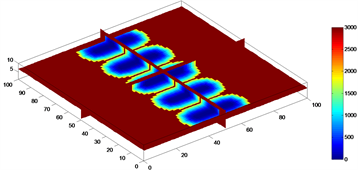(a)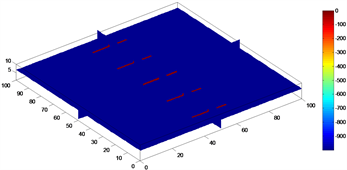(b)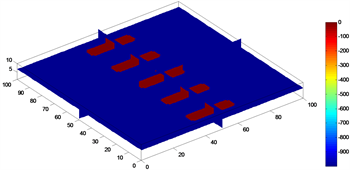(c)

Figure 4. Calculation process of gas drainage volume of multiple fractured horizontal well in tight gas reservoir. (a) The diffusion time (106 s); (b) Drainage area of one month; (c) Drainage area of 10 years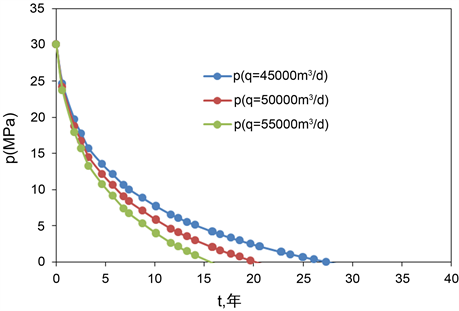Figure 5. Pressure prediction of multiple fractured horizontal well in tight gas reservoir under constant production rate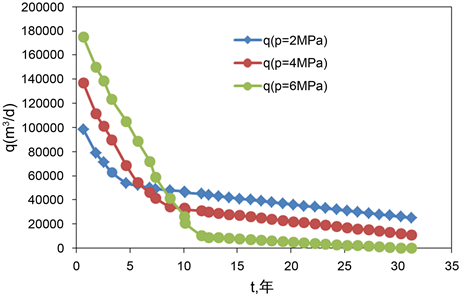Figure 6. Production prediction of multiple fractured horizontal well in tight gas reservoir under constant pressure

5. 结论

1) 常规方法通过计算压力波传播速度来表征生产动态，而快速推进法是通过标记压力波传播时间来开展生产动态分析，具有准确和速度快的特点，这是快速推进法分析致密气井生产动态的核心优势。

2) 致密气藏生产动态分析新方法具有准确性和高效性。基于笛卡尔坐标网格开展压力波前缘传播时间计算，能同时考虑储层的非均质性和井的复杂结构，能较准确地模拟致密气藏的流体流动。并且新模型与数值模拟软件CMG计算结果吻合程度高，计算速度提高了11%，验证了计算模型高效性。

3) 快速推进法(FMM)具有灵活的运用形式，结合几何近似法，分别在定压条件下预测产气量和在定产条件下预测井底流压，根据产量压力动态响应进行合理配产。可快速地估算定压生产和定产生产情况下的井动态特性。快速推进法对构造条件简单的致密气藏生产动态分析，具有很好的应用价值。

  邹才能, 杨智, 何东博, 等. 常规—非常规天然气理论、技术及前景[J]. 石油勘探与开发, 2018, 45(4): 575-587.  孙赞东, 贾承造, 李相方, 等. 非常规油气藏勘探与开发(下册) [M]. 北京: 石油工业出版社, 2011: 865-1092.  孙龙德, 邹才能, 贾爱林, 等. 中国致密油气发展特征与方向[J]. 石油勘探与开发, 2019, 46(6): 1015-1026.  Aderson, D.M., Nobakht, M., Moghadam, S., et al. (2010) Analysis of Production Data from Frac-tured Shale Gas Wells. SPE Unconventional Gas Conference, Pittsburgh, February 2010, SPE-131787-MS. https://doi.org/10.2118/131787-MS  Ozkan, E., Brown, M., Raghavan, R., et al. (2011) Comparison of Frac-tured-Horizontal-Well Performance in Tight Sand and Shale Reservoirs. SPE Reservoir Evaluation & Engineering, 14, 248-259. https://doi.org/10.2118/121290-PA  Stalgorova, E. and Mattar, L. (2012) Practical Analytical Model to Simulate Production of Horizontal Wells with Branch Fractures. The SPE Canadian Unconventional Resources Con-ference, Calgary, October 2012, SPE-162515-MS. https://doi.org/10.2118/162515-MS  Stalgorova, E. and Mattar, L. (2013) Analytical Model for Unconventional Multi-Fractured Composite Systems. SPE Reservoir Evaluation & Engineering, 16, 246-256. https://doi.org/10.2118/162516-PA  Yu, G. and Aguilera, R. (2012) 3D Analytical Modeling of Hydraulic Fracturing Stimulated Reservoir Volume. The SPE Latin America and Caribbean Petroleum Engineering Conference, Mexico City, April 2012, SPE153486, 1-16. https://doi.org/10.2118/153486-MS  Jia, P., Cheng, L.S., Huang, S.J., et al. (2015) Transient Behavior of Complex Fracture Networks. Journal of Petroleum Science and Engineering, 132, 1-17. https://doi.org/10.1016/j.petrol.2015.04.041  王俊超. 基于体积源方法的双重介质渗流模型及其应用[D]: [博士学位论文]. 成都: 西南石油大学, 2015.  孙海. 页岩气藏多尺度流动模拟理论与方法[D]: [博士学位论文]. 东营: 中国石油大学(华东), 2013.  Moinfar, A., Varavei, A., Sepehrnoori, K., et al. (2013) Development of a Coupled Dual Continuum and Discrete Fracture Model for the Simulation of Unconventional Reservoirs. The SPE Res-ervoir Simulation Symposium, The Woodlands, February 2013, SPE-163647-MS. https://doi.org/10.2118/163647-MS  杨军征. 有限体积–有限元方法在油藏数值摸拟中的原理和应用[D]: [博士学位论文]. 北京: 中国科学院, 2011.  Mayerhofer, M.J., Lolon, E.P., Youngblood, J.E., et al. (2006) Inte-gration of Microseismic-Fracture-Mapping Results with Numerical Fracture Network Production Modeling in the Barnett Shale. The SPE Annual Technical Conference and Exhibition, San Antonio, September 2006, SPE-102103-MS, SPE102103, 1-8. https://doi.org/10.2118/102103-MS  Cipolla, C.L., Lolon, E., Mayerhofer, M.J., et al. (2009) Fracture Design Considerations in Horizontal Wells Drilled in Unconventional Gas Reservoirs. The SPE Hydraulic Fracturing Technology Conference, The Woodlands, January 2009, SPE-119366-MS, 1-10. https://doi.org/10.2118/119366-MS  Yang, C. (2013) Application of Fast Marching Method in Shale Gas Res-ervoir Model Calibration. Texas A&M University, College Station.  Sethian, J.A. (1996) A Fast Marching Level Set Method for Monotonically Advancing Fronts. Proceedings of the National Academy of Sciences, 93, 1591-1595. https://doi.org/10.1073/pnas.93.4.1591  Sethian, J.A. (1999) Fast Marching Methods. SIAM Review, 41, 199-235. https://doi.org/10.1137/S0036144598347059  滕柏路, 程林松, 黄世军, 贾振, 艾爽. 基于波前快速推进法的页岩气储层动用预测[J]. 西南石油大学学报(自然科学版), 2016, 38(2): 122-128.  黄世军, 滕柏路, 程林松, 艾爽, 贾振. 基于高精度波前快速推进法的页岩气储层动用分析[J]. 东北石油大学学报, 2014, 38(4): 17-22+118+6.  Kim, J.U., Datta-Gupta, A., Brouwer, R., et al. (2009) Calibration of High-Resolution Res-ervoir Models Using Transient Pressure Data. The SPE Annual Technical Conference and Exhibition, New Orleans, Oc-tober 2009, SPE-124834-MS, 1-17. https://doi.org/10.2118/124834-MS  Xie, J., Gupta, N., King, M.J., et al. (2012) Depth of Investigation and Depletion Behavior in Unconventional Reservoirs Using Fast Marching Methods. SPE Journal, 20, 831-841. https://doi.org/10.2118/154532-MS  Xie, J., Yang, C., Gupta, N., et al. (2012) Integra-tion of Shale Gas Production Data and Microseismic for Fracture and Reservoir Properties Using Fast Marching Method. SPE Journal, 20, 347-359. https://doi.org/10.2118/161357-MS